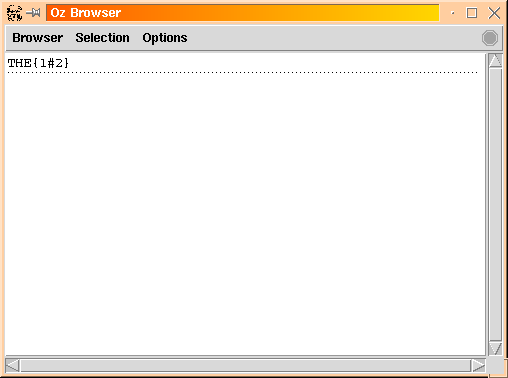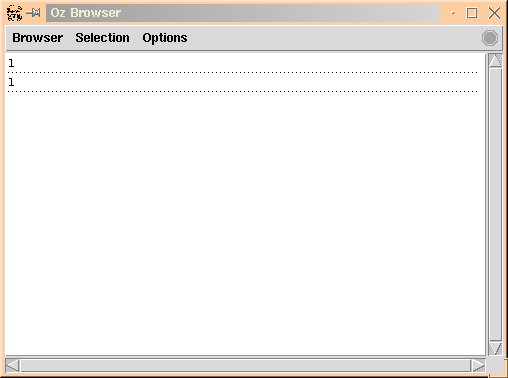## 3.2 Simple Example

As a simple example, lets consider a minuscule English lexicon consisting of the words: `a`, `the`, `man`, `men` and just the information about whether the word is singular or plural. We are going to encode `singular' as the integer 1 and `plural' as the integer 2.

`declare Sing = 1Plur = 2Lexicon = o(a   : [Sing]            the : [Sing Plur]            man : [Sing]            men : [Plur])`

Now, we can represent the ``number'' information of a word `W` by introducing a FD variable `I` and constraining its domain using the information in the lexicon. For example for an occurrence of article `the`, we could write:

`declare THETHE::Lexicon.the`

We can observe this variable in the browser [Pop99] by invoking `{Browse THE}`, and we see:I.e. `THE` is bound to a FD variable whose domain just contains the integers 1 and 2. We can do the same for an occurrence of the word `man`:

`declare MANMAN::Lexicon.man{Browse MAN}`

and we see:Finally we can impose the constraint that the determiner must agree with the noun, i.e. `THE=MAN`, and the display is updated as follows:The ambiguous number information of the determiner has been disambiguated.

Denys Duchier
Version 1.2.0 (20010221)